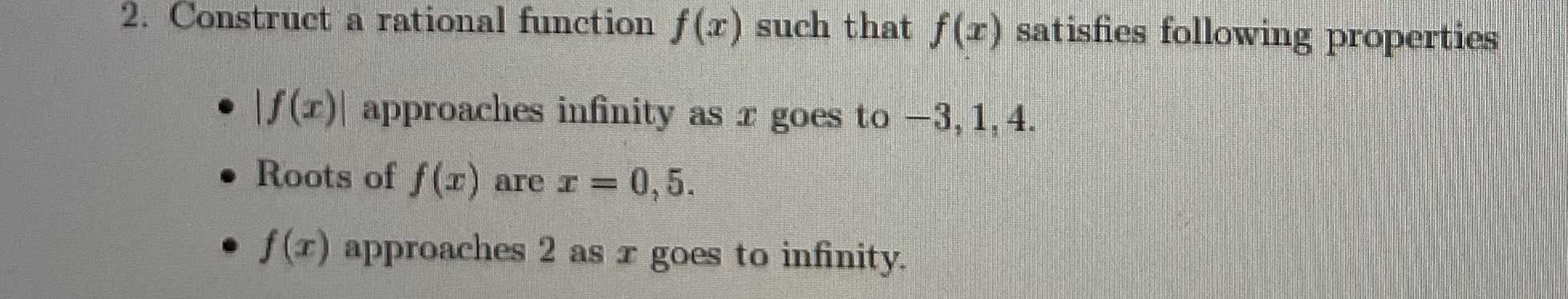### ¿Todavía tienes preguntas de matemáticas?

Pregunte a nuestros tutores expertos
Algebra
Pregunta2. Construct a rational function $$f ( x )$$ such that $$f ( x )$$ satisfies following properties.

$$| f ( x ) |$$ approaches infinity as $$x$$ goes to $$- 3,1,4$$ .

Roots of $$f ( x )$$ are $$x = 0,5$$ .

$$f ( x )$$ approaches $$2$$ as $$x$$ goes to infinity.

$$f(x)= \frac{2x^2(x- 5)}{(x+ 3)(x- 1)(x- 4)}$$